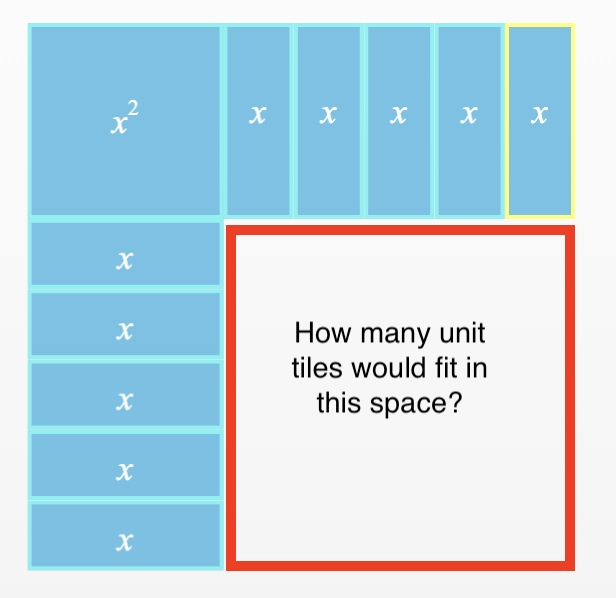### Home > INT2 > Chapter 5 > Lesson 5.2.2 > Problem5-77

5-77.

Suppose you are using algebra tiles to represent each expression below. How many unit tiles would you need to add to build a perfect square?

1. $x^2 + 10x$1. $x^2 – 6x$

$9$ tiles

1. $x^2 + 22x$